﻿ Analyzing Some of the Students' Mistakes through Solving Multiple Choice Questions of Chemistry in Vietnamese High SchoolPublications are Open
Access in this journal
Article Versions
Export Article
• Normal Style
• MLA Style
• APA Style
• Chicago Style
Research Article
Open Access Peer-reviewed

### Analyzing Some of the Students' Mistakes through Solving Multiple Choice Questions of Chemistry in Vietnamese High School

Cao Cu Giac
American Journal of Educational Research. 2019, 7(9), 618-623. DOI: 10.12691/education-7-9-3
Received July 26, 2019; Revised August 28, 2019; Accepted September 08, 2019

### Abstract

Using the results from students' mistakes to make interferential options (confused plans) in multiple choice questions will help teachers analyze the wrong situations and take corrective measures. With the learner's error-based teaching method, students will remember their mistakes and avoid repeating the same mistakes in learning. Multiple choice questions are widely used in the annual National High School Exam in Vietnam. However, in order to assess students' mistakes in the process of organizing teaching and learning in high schools, the design of multiple choice questions should focus on the results of the interferential options. From analyzing the results from wrong situations, the interferential options are strong enough to assess the impact from mistakes.

### 1. Introduction

Many authors have in-depth studies of mistakes in teaching around the world, one of which is Richard M. Felder, North Carolina State University and Rebecca Brent, USA. In these studies, the author has pointed out the ten most important mistakes to be made in the teaching of teachers. One of the most dangerous mistakes he made was that teaching teachers had no clear goals, disrespect and interaction with students, did not grasp the psychology of learners, and did not know if they could make mistakes in the learning process 1.

According to Keith Taber, the different types of mistakes that have a method of impacting students to overcome mistakes are different – Figure 1 2 “Students think and learn in science: views on nature and the development of learners' ideas”.

• Figure 1. Keith Taber's model of error analysis in teaching

Following Keith Taber's model above as well as Andy Chandler - Grevatt' s conception, learners' mistakes are mainly due to four reasons: lack of knowledge, lack of connection, misunderstanding or misconceptions. “The difference between a misunderstanding and a misconception is that misunderstandings often arise in the teaching and learning process. Meanwhile, misconceptions have developed outside the classroom. They are often culturally formed and strongly organized” 3.

In the same view, James H. McMillan shows why erroneous analysis is an essential part of effective learning and how it can be used by teachers to promote student learning 4.

The page "www.matrix.edu.au" also pointed out the eight major mistakes students make in the HSC exam in Australia's chemistry, including common mistakes the same as Vietnamese students, such as writing the wrong chemical equation, not mastering the knowledge of solubility, ... 5.

According to Andy Chandler on the famous "www.educaton in chemistry" page on Chemistry teaching, experienced teachers will discover common mistakes of learners in chemical learning. However, they do not always recognize the reasons for mistakes and remedies are often an important issue. Pinchpoint method will show the causes of mistakes of learners and allow differentiating different groups of mistakes. In addition, students can apply difficult concepts or skills more confidently into new situations 6.

In 2013, in the set of book about introductory free questions and answers for the AP exam (Advanced Placement), the authors gave an overview of each self-answer question and how students performed on the those answers. For each question that requires students to point out fundamental mistakes 7.

In Vietnam, there have been some studies by Cao Cu Giac, Nguyen Xuan Truong and Hoang Thanh Phong on mistakes of students during solving high school chemistry exercises, which have pointed out some mistakes when solving inorganic chemistry exercises 8. Within the scope of this paper, we will analyze some students' mistakes through high school multiple choice exercises.

### 2. Analyze Some Mistakes in the Process of Solving the Multiple Choice Questions of Chemistry

Using the results from students' mistakes to make interferential options in multiple choice questions, will help teachers analyze the wrong situations that occur and take corrective measures. With students' mistake-based teaching methods, students will remember their mistakes and avoid repeating the same mistakes in learning.

Example 1: Acyclic compound X has a molecular formula of C4H9NO2. Given 10.3 grams of X to react sufficiently with NaOH solution to produce a gas Y and solution Z. Y gas is denser than air, causing moist purple litmus paper to turn green. Solution Z may discolor bromine solution. Boiling up the Z solution to get m gram of anhydrous salt. The value of m is

A. 8.2 B. 9.4 C. 9.6 D. 10.8

Thought Process: When solving this exercise, students often make some mistakes through the following situations:

Mistake situation 1: Students who do not pay attention to the fact that Y gas is heavier than air should assume that X is ammonium salt, deduce the structural formula of X is CH2=CH–CH2–COONH4. The reaction equation:

CH2=CH–CH2–COONH4 + NaOH

0.1 mol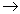CH2=CH–CH2– COONa + NH3 + H2O

0.1 mol

Mass of salt: m = 0.1 x 108 = 10.8 (g)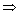The wrong answer is D.

Mistake situation 2: Students do not consider solution Z to be able to discolor bromine solution, so X has a structural formula of: CH3COOCH2CH2NH2.

CH3COOCH2CH2NH2 + NaOH

0.1 molCH3COONa + HO-CH2-CH2-NH2

0.1 mol

Mass of salt: m = 0.1 x 82 = 8.2 (g)The wrong answer is A.

The correct solution for this exercise is:

Because X + NaOHY (gas)

Y is denser than air (Mair = 29 g/mol) so Y cannot be NH3X has the structure formula of CH2=CHCOONH3CH3 (because Z is capable of discoloring Br2 solution, Z has double bonds C=C):

CH2=CHCOONH3CH3 + NaOH

0.1 molCH2 = CHCOONa + CH3NH2 + H2O

0.1 mol

Number of moles C4H9NO2 = 10.3/103 = 0.1 molmZ = 0.1.94 = 9.4 (g)The correct answer is B.

Example 2: Add 5.6 g of iron powder into the excess AgNO3 solution, stir until the reaction is complete, how many grams of solids are obtained?

A. 24.2 B. 21.6 C. 32.4 D. 10.8

Thought Process: The excess AgNO3 solution so Fe is oxidized completely to Fe(NO3)3.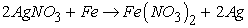(1)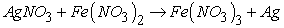(2)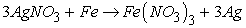(3)

From (3) →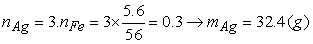Answer is C.

Mistake situation 1: If students just stop at the chemical equation of reaction (1) and calculate because they do not know or forget that excess AgNO3 can further oxidize Fe(NO3)2 to Fe(NO3)3. From (1)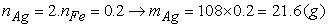The interferential option is B.

Mistake situation 2: If students still write the chemical equation for the reaction (3) but does not balance, leads to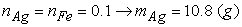The interferential option is D.

Mistake situation 3: If students think that solids are Fe(NO3)3 salts and the number of moles is equal to the number of moles of Fe so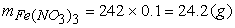The interferential option is A.

Example 3: Which of the following substance has the lowest boiling point?

Thought Process: Two options B and C are built similarly to the option A, which are both benzenediol making it difficult for students to distinguish. Option D has two groups in the ortho position of each other like option A also has good interferential effects. Thus, the similarity is often confusing for students, if they do not understand the influence of intermolecular and intra-molecular hydrogen bonds on the boiling point of the substance, it is easy to be attracted by the above interferential options.

### 3. Results and Discussion

In order to evaluate the impact of the erroneous analysis in the process of solving the multiple choice questions of chemistry that positively affect the quality of teaching and learning chemistry at high schools in Vietnam, we organized to teach experimental groups and the control groups to test and analyze the scores, obtaining the following results (Table 1).

Experimental results are handled by mathematical statistical methods 9, 10 as follows: (i) Make a table of distribution of frequencies, cumulative frequency; (ii) Graph the cumulative line from the cumulative frequency distribution table; (iii) Calculate the typical parameters.• Average value: Typical for data concentration.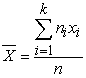where ni is the frequency of xi values and n is the number of experimental students.

Variance S2 and standard deviation S: These are parameters that measure the degree of dispersion of data around the mean value.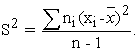The smaller the S value, the less dispersed the data.

Standard error of m: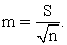The value of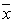will vary in the segment [- m;+ m].

Coefficient of variation of V: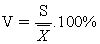- When two tables of data of two groups have equivalent values, based on the standard deviation value S, the group with small S is the better quality group.

- When the two tables of data of the two groups have different values, compare the value of V. The group with small value of V is the group with more uniform quality.

To confirm the difference between 2 values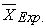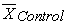is significant with significance level α, we use the t - Student test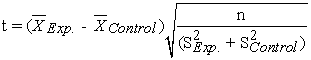Choose α from 0.01 to 0.05, look at the student distribution table to find the value of t(α, k) with free deviation k = 2n - 2.

- If t ≥ t(α, k) then the difference betweenandis significant with significance level α.

- If t < t(α, k) then the difference betweenandis not significant enough with significance level α.

• Figure 2. Percent of students scoring Xi or below (Lesson 1)
• Figure 3. Percent of students scoring Xi or below (Lesson 2)

Through the results of pedagogical experiment, we found that the experimental results of the experimental group were higher than the control group, shown in:

(1) The percentage of students who achieved a low level in the experimental group is always lower than the control group and vice versa, the percentage of students achieving a high and average of the experimental group is higher than the control group.

(2) The graph of the cumulative lines of experimental group is on the right and below the graph of the control lines of the control group.

(3) The mean score of experimental group was higher than the control group.

(4) Use the student-test for 2 tests:

Test 1: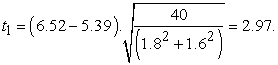In the Student distribution table, take α = 0.05 where k = 40 x 2 - 2 = 78 → tk,α = 2.640.

Thus, t1 > tk,αthe difference betweenandis significant.

Test 2: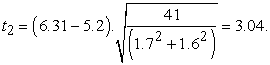In the Student distribution table, take α = 0.05 where k = 41 x 2 - 2 = 80 → tk,α = 2.639.

Thus,, t2 > tk,αthe difference betweenandis significant.

### 4. Conclusion

Multiple-choice questions are widely used in the annual national high school exams in Vietnam. However, to assess the mistakes of students in the process of organizing teaching and learning in high schools, the design of multiple choice questions should focus on the results of the interference options. The analysis of the results from the erroneous situations will make the interference options strong enough to assess the effects from the mistakes.

### Acknowledgements

This research is funded by the Centre for Research – Entrepreneurship Innovation, Vinh University, under contract No 04/2018/TTNC-KNST. We sincerely thank that support.

### References

  https://www.researchgate.net/profile/Richard_Felder. In article  Keith Taber (2014). Student thinking and learning in science. New York, NY: Routledge. In article View Article  Andy Chandler – Grevatt (2018), How to make the most of mistakes. Routledge Publisher In article  James H. McMillan (2017). Using Students' Assessment Mistakes and Learning Deficits to Enhance Motivation and Learning. Routledge Publisher. In article View Article  www.matrix.edu.au. In article  www.educaton in chemistry. In article  Student Performance Q&A: 2013 AP Chemistry Free - Response Question Chief Reader, Larry Funk of Wheaton College, Wheaton, Illinoi-USA. In article  Cao Cu Giac, Hoang Thanh Phong (2010), “Analyze common mistakes by guiding students to solve chemistry exercises”, Journal of Chemistry and Application (Topical number of scientific research results), Vietnam, No 3/2010 pp.6-9. In article  Eichler A., Zapata-Cardona L. (2016). Empirical Research in Statistics Education. In: Empirical Research in Statistics Education. ICME-13 Topical Surveys. Springer, Cham. In article View Article  Schau, C., Stevens, J., Dauphinee, T. L., & Vecchio, A. D. (1995). The development and validation of the survey of attitudes toward statistics. Educational and Psychological Measurement, 55(5), 868-875. In article View ArticleThis work is licensed under a Creative Commons Attribution 4.0 International License. To view a copy of this license, visit http://creativecommons.org/licenses/by/4.0/